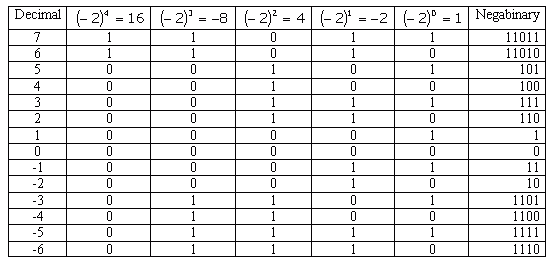Subject: arithmetic with base -2 Name: yuva Who are you: Student can we do arithmetic ,base -2. If yes, how and if not, why. Plz help me with this Hi Yuva. Yes, it can be done, but it's far from convenient. In fact, some experimental computers once ran using this notation, which is called "negabinary" notation. The most useful aspect of this numbering is that you don't need a negative sign to represent negative or positive numbers. Here are some integers in decimal, expansions, and negabinary:So you can see that the counting sequence is rather unusual, as are the observations using this notation: 110 + 111 = 101 110 - 111 = 11 110110 = 100 1000 / 10 = 100 with perhaps the last line being quite surprising. There is more information on negabinary here on Wikipedia. Stephen La Rocque.>